# Simple Example of A* Pathfinding: A maze with no obstacles

suggest change

Let’s say we have the following 4 by 4 grid: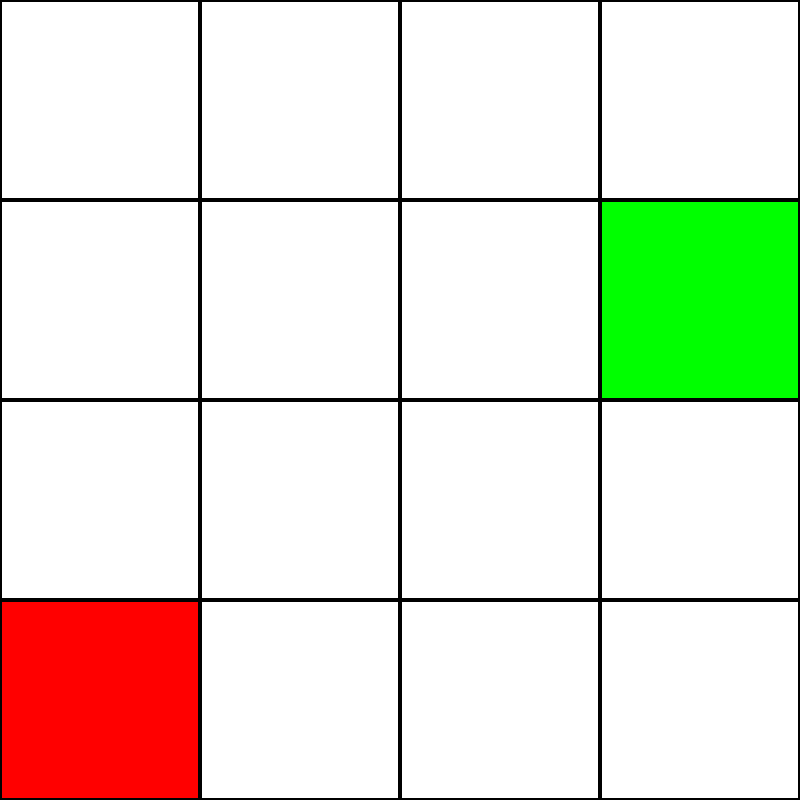Let’s assume that this is a maze. There are no walls/obstacles, though. We only have a starting point (the green square), and an ending point (the red square). Let’s also assume that in order to get from green to red, we cannot move diagonally. So, starting from the green square, let’s see which squares we can move to, and highlight them in blue: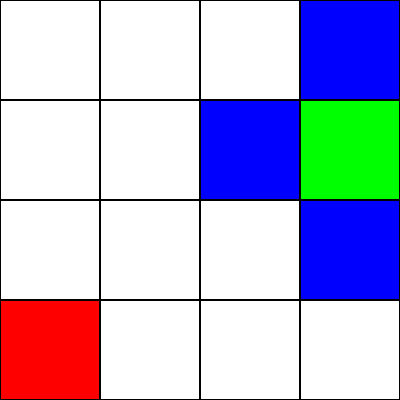In order to choose which square to move to next, we need to take into account 2 heuristics:

1. The “g” value - This is how far away this node is from the green square.
2. The “h” value - This is how far away this node is from the red square.
3. The “f” value - This is the sum of the “g” value and the “h” value. This is the final number which tells us which node to move to.

In order to calculate these heuristics, this is the formula we will use: `distance = abs(from.x - to.x) + abs(from.y - to.y)`

This is known as the “Manhattan Distance” formula.

Let’s calculate the “g” value for the blue square immediately to the left of the green square: `abs(3 - 2) + abs(2 - 2) = 1`

Great! We’ve got the value: 1. Now, let’s try calculating the “h” value: `abs(2 - 0) + abs(2 - 0) = 4`

Perfect. Now, let’s get the “f” value: `1 + 4 = 5`

So, the final value for this node is “5”.

Let’s do the same for all the other blue squares. The big number in the center of each square is the “f” value, while the number on the top left is the “g” value, and the number on the top right is the “h” value: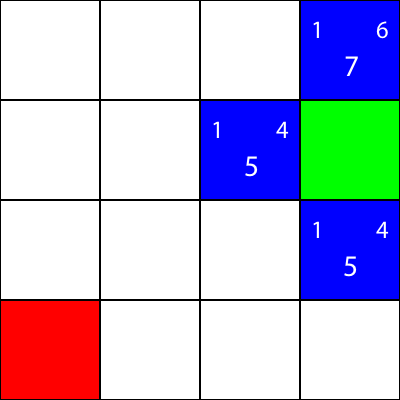We’ve calculated the g, h, and f values for all of the blue nodes. Now, which do we pick?

Whichever one has the lowest f value.

However, in this case, we have 2 nodes with the same f value, 5. How do we pick between them?

Simply, either choose one at random, or have a priority set. I usually prefer to have a priority like so: “Right > Up > Down > Left”

One of the nodes with the f value of 5 takes us in the “Down” direction, and the other takes us “Left”. Since Down is at a higher priority than Left, we choose the square which takes us “Down”.

I now mark the nodes which we calculated the heuristics for, but did not move to, as orange, and the node which we chose as cyan: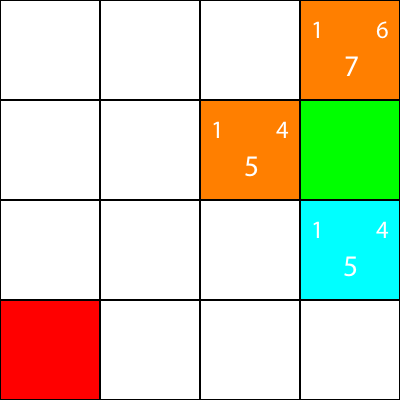Alright, now let’s calculate the same heuristics for the nodes around the cyan node: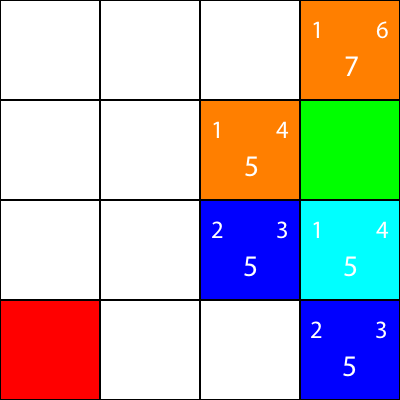Again, we choose the node going down from the cyan node, as all the options have the same f value: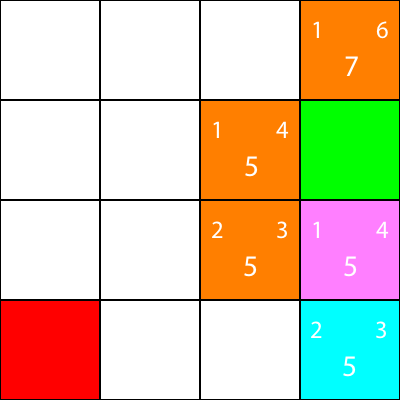Let’s calculate the heuristics for the only neighbour that the cyan node has: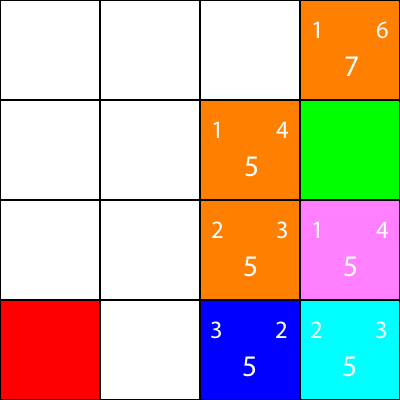Alright, since we will follow the same pattern we have been following: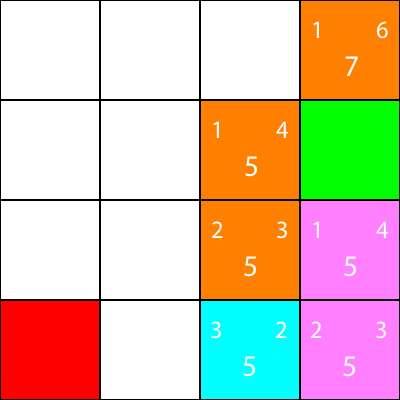Once more, let’s calculate the heuristics for the node’s neighbour: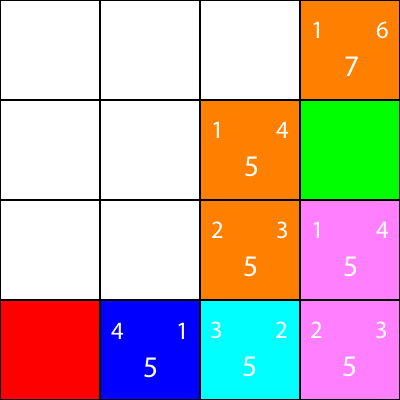Let’s move there: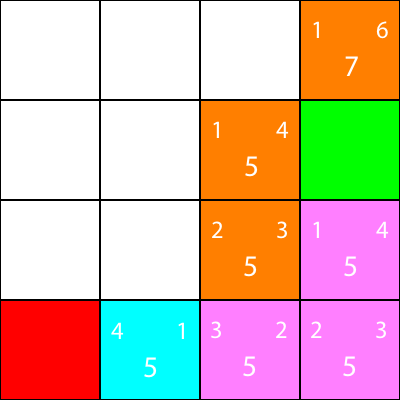Finally, we can see that we have a winning square beside us, so we move there, and we are done.